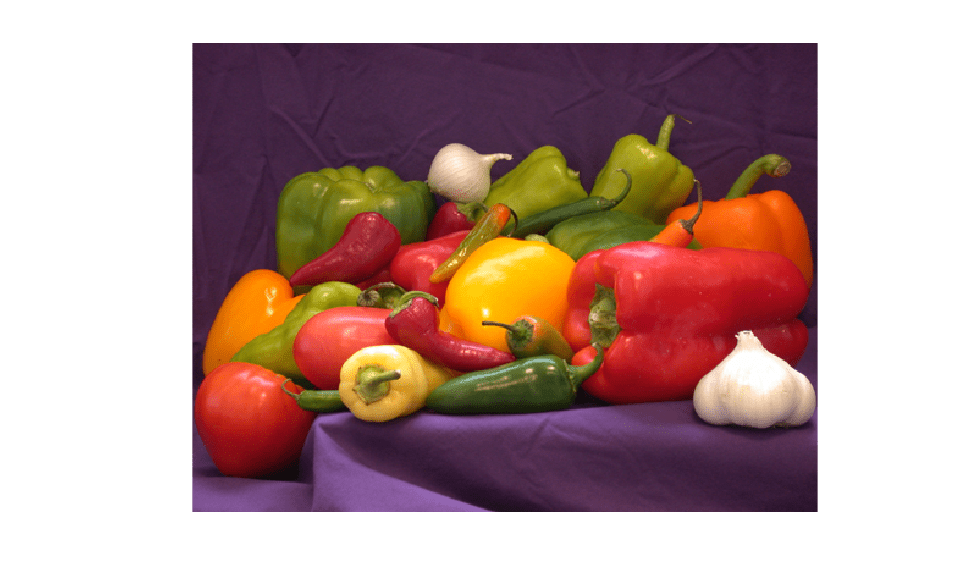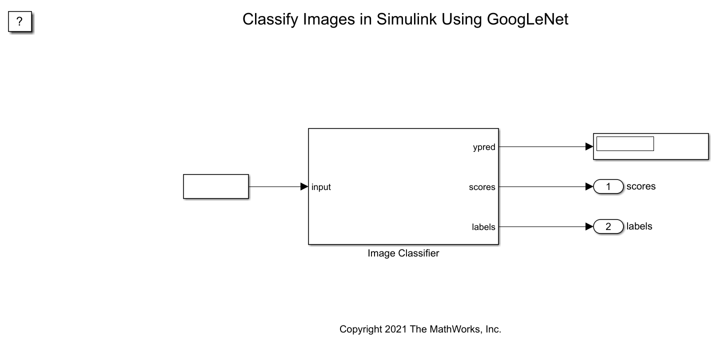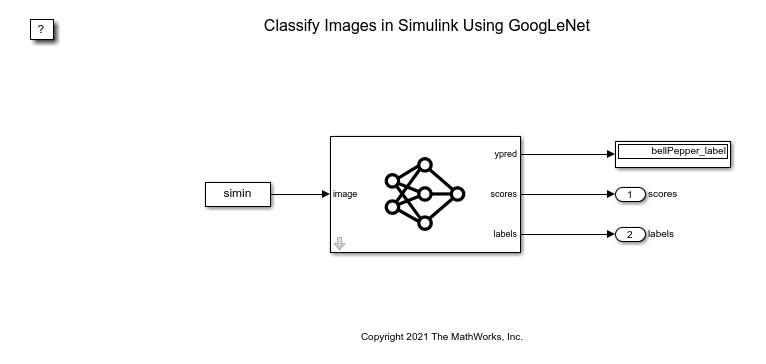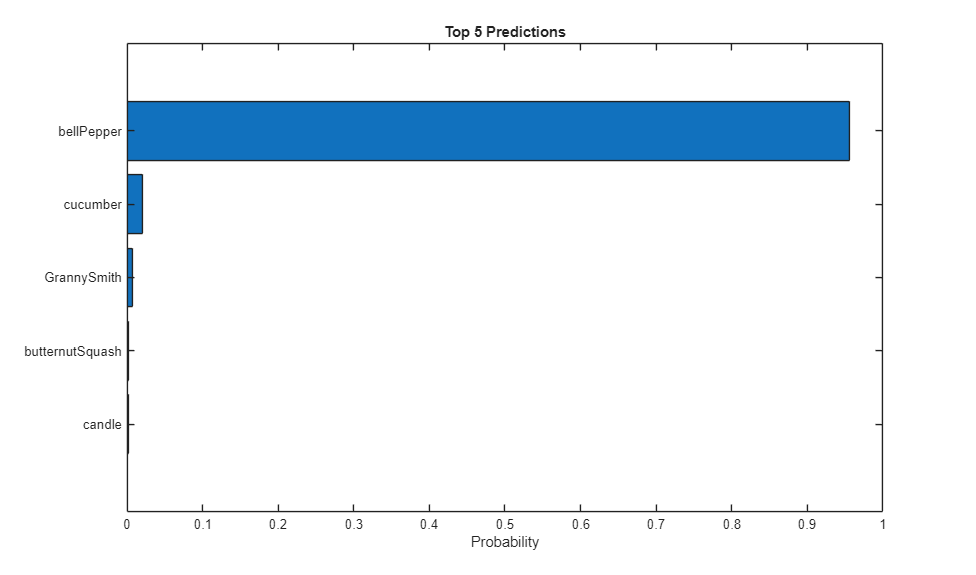This example shows how to classify an image in Simulink® using the `Image Classifier` block. The example uses the pretrained deep convolutional neural network GoogLeNet to perform the classification.

GoogLeNet has been trained on over a million images and can classify images into 1000 object categories (such as keyboard, coffee mug, pencil, and many animals). The network has learned rich feature representations for a wide range of images. The network takes an image as input, and then outputs a label for the object in the image together with the probabilities for each of the object categories.

```net = googlenet; inputSize = net.Layers(1).InputSize; classNames = net.Layers(end).ClassNames; numClasses = numel(classNames); disp(classNames(randperm(numClasses,10))) ```
``` {'speedboat' } {'window screen'} {'isopod' } {'wooden spoon' } {'lipstick' } {'drake' } {'hyena' } {'dumbbell' } {'strawberry' } {'custard apple'} ```

Read and show the image that you want to classify.

```I = imread('peppers.png'); figure imshow(I) ```To import this data into the Simulink model, specify a structure variable containing the input image data and an empty time vector.

```simin.time = []; simin.signals.values = I; simin.signals.dimensions = size(I); ```

The Simulink model for classifying images is shown. The model uses a `From Workspace` block to load the input image, an `Image Classifier` block from the Deep Neural Networks library that classifies the input, and `Display` block to show the predicted output.

```model = 'googlenet_classifier'; open_system(model); ```### Run the Simulation

To validate the Simulink model, run the simulation.

```set_param(model,'SimulationMode','Normal'); sim(model); ```

The network classifies the image as a bell pepper.### Display Top Predictions

Display the top five predicted labels and their associated probabilities as a histogram. Because the network classifies images into so many object categories, and many categories are similar, it is common to consider the top-five accuracy when evaluating networks. The network classifies the image as a bell pepper with a high probability.

```scores = yout.signals(1).values(:,:,1); labels = yout.signals(2).values(:,:,1); [~,idx] = sort(scores,'descend'); idx = idx(5:-1:1); scoresTop = scores(idx); labelsTop = split(string(labels(idx)),'_'); labelsTop = labelsTop(:,:,1); figure imshow(I) title(labelsTop(5) + ", " + num2str(100*scoresTop(5) + "%")); figure barh(scoresTop) xlim([0 1]) title('Top 5 Predictions') xlabel('Probability') yticklabels(labelsTop) ```